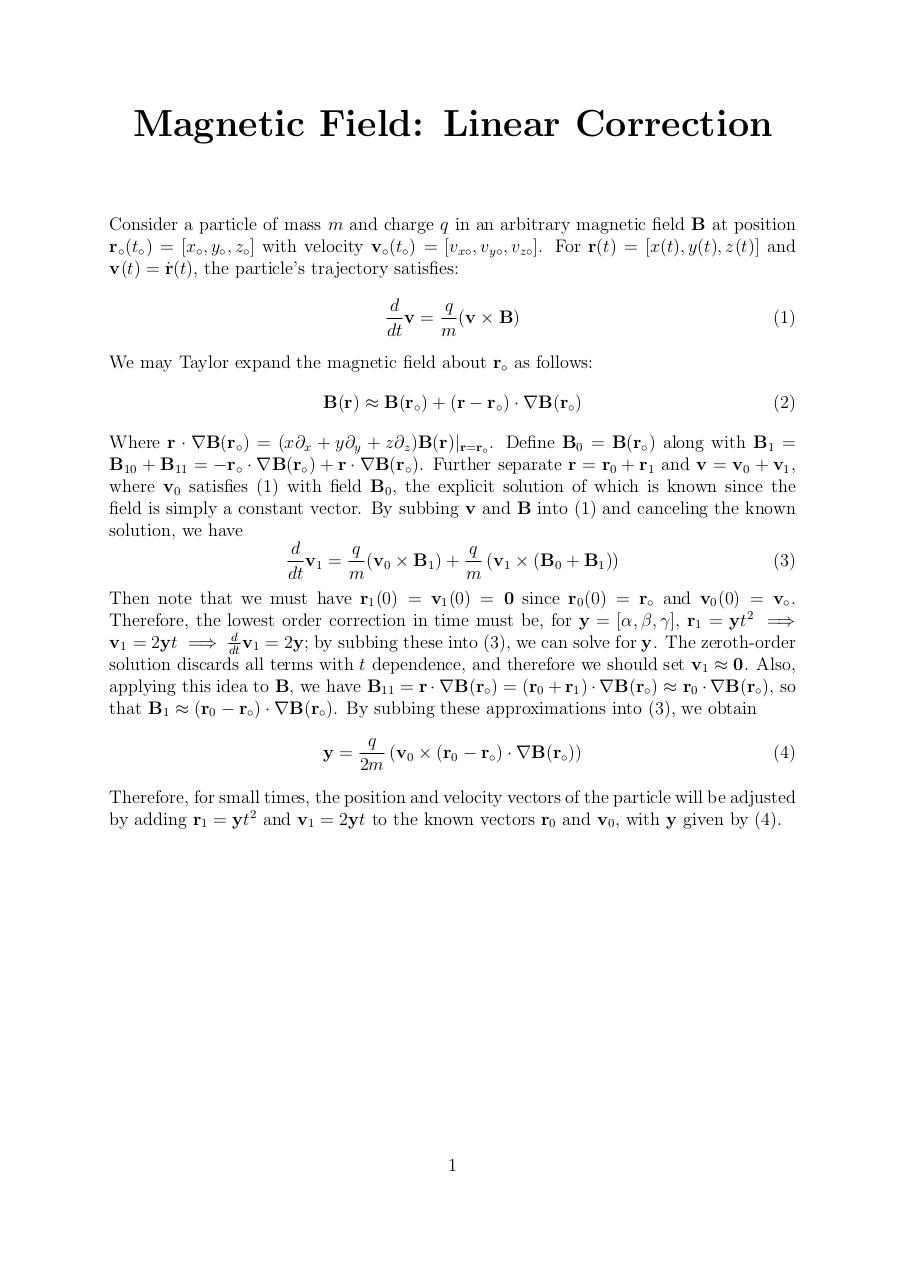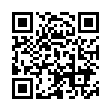# B correction .pdf

### File information

Original filename: B_correction.pdf

This PDF 1.5 document has been generated by TeX / MiKTeX pdfTeX-1.40.12, and has been sent on pdf-archive.com on 09/06/2013 at 18:16, from IP address 108.170.x.x. The current document download page has been viewed 985 times.
File size: 90 KB (1 page).
Privacy: public file

B_correction.pdf (PDF, 90 KB)

### Document preview

Magnetic Field: Linear Correction
Consider a particle of mass m and charge q in an arbitrary magnetic field B at position
r◦ (t◦ ) = [x◦ , y◦ , z◦ ] with velocity v◦ (t◦ ) = [vx◦ , vy◦ , vz◦ ]. For r(t) = [x(t), y(t), z(t)] and
v(t) = r˙ (t), the particle’s trajectory satisfies:
q
d
v = (v × B)
dt
m

(1)

We may Taylor expand the magnetic field about r◦ as follows:
B(r) ≈ B(r◦ ) + (r − r◦ ) · ∇B(r◦ )

(2)

Where r · ∇B(r◦ ) = (x∂x + y∂y + z∂z )B(r)|r=r◦ . Define B0 = B(r◦ ) along with B1 =
B10 + B11 = −r◦ · ∇B(r◦ ) + r · ∇B(r◦ ). Further separate r = r0 + r1 and v = v0 + v1 ,
where v0 satisfies (1) with field B0 , the explicit solution of which is known since the
field is simply a constant vector. By subbing v and B into (1) and canceling the known
solution, we have
d
q
q
v1 = (v0 × B1 ) + (v1 × (B0 + B1 ))
(3)
dt
m
m
Then note that we must have r1 (0) = v1 (0) = 0 since r0 (0) = r◦ and v0 (0) = v◦ .
Therefore, the lowest order correction in time must be, for y = [α, β, γ], r1 = yt2 =⇒
v1 = 2yt =⇒ dtd v1 = 2y; by subbing these into (3), we can solve for y. The zeroth-order
solution discards all terms with t dependence, and therefore we should set v1 ≈ 0. Also,
applying this idea to B, we have B11 = r · ∇B(r◦ ) = (r0 + r1 ) · ∇B(r◦ ) ≈ r0 · ∇B(r◦ ), so
that B1 ≈ (r0 − r◦ ) · ∇B(r◦ ). By subbing these approximations into (3), we obtain
y=

q
(v0 × (r0 − r◦ ) · ∇B(r◦ ))
2m

(4)

Therefore, for small times, the position and velocity vectors of the particle will be adjusted
by adding r1 = yt2 and v1 = 2yt to the known vectors r0 and v0 , with y given by (4).

1Use the permanent link to the download page to share your document on Facebook, Twitter, LinkedIn, or directly with a contact by e-Mail, Messenger, Whatsapp, Line..

Use the short link to share your document on Twitter or by text message (SMS)

#### HTML Code

Copy the following HTML code to share your document on a Website or Blog

#### QR Code### Related keywords